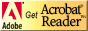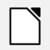Pages created and updated by Terry Sturtevant Date Posted: May 12, 2017

Contact me if you have any other suggestions for examples to include.
For what it's worth, I work on a Linux system using TeX Live , which is also available for Windows, but many students may work with these examples on Windows systems using MikTeX or some other flavour, , and some work on Macs . That's why I have three versions of the source files. (Any one will work fine with LATEX, but some text editors will have a problem with the wrong version.)

# LATEX by example

LATEX can be learned somewhat like HTML; by looking at some simple examples of LATEX source code and the resulting output, even beginners can quickly get the idea of how various things work. The examples use pdflatex to produce the output, since there are many features available in pdf documents. Since these examples use complete source files, some of which are from real documents of mine, sometimes certain elements, (such as math mode, figures and tables), will show up in several examples, even though they don't fall under that specific topic heading. By the way, the proper way to write LATEX as straight text is LaTeX.

## A note about these LATEX file examples

I use pdflatex to produce the output for all of my work, so that is how these examples are set up. For each of the examples, if you place all of the files (for whichever operating system you prefer) from one example in the same directory, then if you have pdflatex installed, you should be able to produce the pdf output shown. For the first example, that would mean typing
```pdflatex simple
```
from the directory in which the files are placed. (You don't need to include the '.tex' extension.)

If you get errors indicating pdflatex cannot be found, try typing
```PATH=%PATH%;"C:\Program Files\MiKTeX 2.9\miktex\bin"
```
or
```PATH=%PATH%"C:\Program Files\MiKTeX 2.9\miktex\bin;"
```
at the command prompt after installing MiKTeX.

Here's an ogg video showing how a document is taken from a text file to a pdf file. (The example is on a Linux system, but the process should be very similar on other platforms.)

## Simple LATEX file examples

### Example with title information

Note that in this case, you can see how maketitle works differently in an article and a report.
A line beginning with a percent sign is a comment; it is ignored by LATEX but may be useful to you.

## LATEX examples of document structure elements

### Examples with an abstract

You could make the abstract a section like any other, but the abstract environment handles it more like the way professional journals do.
Note the differences between an article and a report.

### Example using the LATEX input command, which allows breaking up a single large document into several smaller chunks.

This simplifies editing large documents, especially when different parts of the document are logically distinct, such as chapters in a book.
Another useful occasion for this is when you have common definitions which will be used for many documents, such as this macro file example.

## LATEX examples of math mode

### Illustration of various LATEX math modes.

This also shows how a numbered equation can be referenced elsewhere in a document. (The tilde character is used in LATEX to produce a non-breaking space; i.e. a space between two things where a line break will not be allowed.)

## LATEX examples with other elements; lists, tables, figures, etc.

### LATEX list example

This includes both ordered and unordered lists.

### LATEX table example

There are entire books written about typesetting tables, not only in LATEX, but this will give a brief introduction to the basics.

### LATEX figure example

If you're trying to get graphs from Excel into LATEX, do this:
1. Select the graph and copy it into the clipboard.
2. Open Paint and paste it in.
3. Save the file as a PNG.
4. Rename the file so that png is in lower case, not upper case. ( LATEX is case sensitive.)
5. Import as in the following example.
In order to make the graph look better, turn off all background shading, and don't put a legend, titles, or axis labels on the graph. Adding all of the text in LATEX will make the result look a lot better.

## Creating references and bibliographies in LATEX

### LATEX with reference

If you're doing much of this, BibTeX is the way to go. Sometime I may add more here about it if I get the time. If you just have to do an occasional reference, the following will get you through.

## Importing spreadsheets from other applications into LATEX

### Example using Excel2LaTeX

This is a plugin for Excel to simplify producing LATEX tables from spreadsheet tables. Note that it doesn't get all of the lines from the original, and which ones it gets depends on what part of the spreadsheet you select.

### Example using Calc2LaTeX for OpenOffice

Here is a plugin to simplify producing LATEX tables from OpenOffice spreadsheet tables. In contrast to the previous example, this one tends to get more lines than in the original spreadsheet.

## Example using the hyperref package :

I've taken the example above for many math features and added one line to the file. Now all of the labels in the file become hyperlinks, so that in the PDF output, you can click on references to tables and figures and the viewer will jump to the link. (See the following example for more.)

## Another example using the hyperref package :

I've taken the example above and changed all of the equations to use the equation environment so they can have labels. The last page of the document has links to each equation to show them working.

# Other LATEX resources

ΣquPlus This is an interesting site with actual LaTeX code for lots of equations which you can cut and paste into your documents.

Online Postscript to PDF converter For those rare occasions when you want to make a figure from a package that can't produce .PNG or .JPG output. Select a postscript printer (even if you don't actually have one), and print to a file rather than to the printer. Then use the converter to turn the output into a .PDF file which you can incorporate into a pdflatex document.

Cambridge University Help on LaTeX Commands

More Cambridge university LaTeX help Also nicely indexed

Getting Started with LaTeX This one displays everything on HTML pages, so it doesn't need a PDF viewer to see the examples of output

LaTeX Tutorial in PDF format This is another one that starts with the basics

The Not So Short Introduction to LaTeX Starts from the beginning

pdflatex information Gives a few useful tips on using the hyperref package

PDF LaTex by example Examples include lots of bells and whistles if you really want to push the envelope

Deadly sins; The most severe mistakes in using LaTeX2e gives some tips on the best way to do things like adjust line spacing in LaTeX

User's guide for the amsmath package shows the best way to typeset many things (particularly equations) in LaTeX

The LaTex style file for the Canadian Journal of Physics

Excel2LaTeX:a package to convert Excel tables to LaTeX tables

Get Crimson Editor, a freeware editor for Windows with syntax highlighting for several languages including LaTeX

Here are some bits of cut-and-paste HTML code for getting the LATEX logo in the headings and within regular paragraphs.

## LATEX

### LATEX

#### LATEX

##### LATEX
###### LATEX

LATEX

Here's the stylesheet you need for the LaTeX logos above.
latex.css
Date Posted: February 13, 2007

## ResourcesIf you need to update a browser, you might try Firefox which is
• free
• open source
• available for several platformsSince this page uses cascading style sheets for its layout, it will look best with a browser which supports the specifications as fully as possible.

If you are looking for an office package, with a word processor, spreadsheet, etc., you might try LibreOffice which is
• free
• open source
• available for several platformsGo to the main page for the Department of Physics and Computer Science.

Wilfrid Laurier University